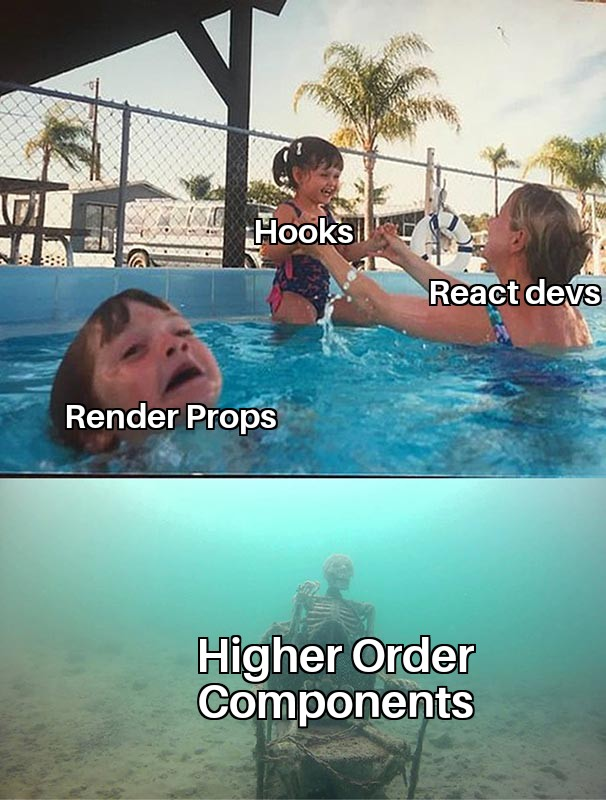# React 设计模式和思想

June 21, 2018

React 社区在近几年的发展过程中诞生了很多有趣的组件设计 "模式"，通过合理的运用这些模式可以让我们写出更好用的 React 组件。``````const Tab = ({ value, active, onChange, children }) => (
<div className={active && 'active'} onClick={evt => onChange(value, evt)}>
{children}
</div>
)
``````

``````class Index extends React.Component {
state = {
value: 'a'
}

handleChange = value => {
this.setState({ value })
}

render() {
return (
<div>
<Tab value="a" active={this.state.value === 'a'} onChange={this.handleChange}>
Tab A
</Tab>
<Tab value="b" active={this.state.value === 'b'} onChange={this.handleChange}>
Tab B
</Tab>
<Tab value="c" active={this.state.value === 'c'} onChange={this.handleChange}>
Tab B
</Tab>
</div>
)
}
}
``````

## 复合组件 Compound Components

``````const Tabs = ({ value, onChange, onClick, children }) =>
React.Children.map(children, el => {
const { value: childValue } = el.props
return React.cloneElement(el, {
onChange,
onClick: onChange,
active: value === childValue,
value: childValue
})
})
``````

``````class Index extends React.Component {
state = {
value: 'Tab A'
}

handleChange = value => {
this.setState({ value })
}

render() {
return (
<Tabs value={this.state.value} onChange={this.handleChange}>
{['Tab A', 'Tab B'].map(tab => (
<Tab value={tab} key={tab}>
{tab}
</Tab>
))}
</Tabs>
)
}
}
``````

• Tab 组件只能作为 Tabs 的直系子组件，不能被其他组件包裹
• Tabs 组件只能包含 Tab 组件

## Context

``````const initialValue = {
value: '',
onChange() {}
}

const TabsContext = React.createContext(initialValue)

const Tab = ({ value, children }) => (
<TabsContext.Consumer>
{ctx => (
<div className={ctx.value === value && 'active'} onClick={evt => ctx.onChange(value, evt)}>
{children}
</div>
)}
</TabsContext.Consumer>
)

const Tabs = ({ value, onChange, children }) => (
<TabsContext.Provider value={{ value, onChange }}>{children}</TabsContext.Provider>
)
``````

``````class Index extends React.Component {
state = {
value: 'Tab A'
}

handleChange = value => {
this.setState({ value })
}

render() {
return (
<Tabs value={this.state.value} onChange={this.handleChange}>
{['Tab A', 'Tab B'].map(tab => (
<span key={tab}>
<Tab value={tab}>{tab}</Tab>
</span>
))}
</Tabs>
)
}
}
``````

## Render Props

``````const Tab = ({ value, active, onChange, children, render }) => {
if (typeof render === 'function') {
return render({ value, active, onChange })
}
return (
<div className={active && 'active'} onClick={evt => onChange(value, evt)}>
{children}
</div>
)
}
``````

``````class Index extends React.Component {
state = {
value: 'Tab A'
}

handleChange = value => {
this.setState({ value })
}

render() {
return (
<Tabs value={this.state.value} onChange={this.handleChange}>
{['Tab A', 'Tab B'].map(tab => (
<Tab
value={tab}
render={({ value, active, onChange }) => (              <li className={active && 'active'} onClick={evt => onChange(value, evt)}>                <span>{tab}</span>              </li>            )}            key={tab}
/>
))}
</Tabs>
)
}
}``````

## 高阶组件 HOC(Higher Order Component)

``````const NavBar = ({ user }) => (
<nav>
User: <span>{user ? user.name : 'fetching...'}</span>
</nav>
)
``````

``````const withUser = WrappedComponent =>
class HocWithUser extends React.Component {
state = {
user: null
}

componentDidMount() {
fetchUser().then(user => {
this.setState({ user })
})
}

render() {
return <WrappedComponent {...this.porps} user={this.state.user} />
}
}
``````

``````const NavBar = ({ user }) => (
<nav>
User: <span>{user ? user.name : 'fetching...'}</span>
</nav>
)
const NavBarWithUser = withUser(NavBar)

class Index extends React.Component {
render() {
return <NavBarWithUser />
}
}
``````

1. `withUser` 返回的组件在 `ReactDevTools` 里显示的名称都是相同的 `HocWithUser`，这会影响我们的 Debug 效率
2. 调用 `withUser` 返回的组件是无法访问到 `WrappedComponent` 的静态属性，这显然是不行的

``````const withUser = WrappedComponent =>
class HocWithUser extends React.Component {
static displayName = `withUser(\${WrappedComponent.displayNanme || WrappedComponent.name || 'Component'})`
state = {
user: null
}

componentDidMount() {
fetchUser().then(user => {
this.setState({ user })
})
}

render() {
return <WrappedComponent {...this.porps} user={this.state.user} />
}
}``````

``````import hoistStatics from 'recompose/hoistStatics'

const withUser = hoistStatics(
WrappedComponent =>
class HocWithUser extends React.Component {
static displayName = `withUser(\${WrappedComponent.displayNanme || WrappedComponent.name || 'Component'})`

state = {
user: null
}

componentDidMount() {
fetchUser().then(user => {
this.setState({ user })
})
}

render() {
return <WrappedComponent {...this.porps} user={this.state.user} />
}
}
)
``````

## 在 controlled 和 uncontrolled 之间切换

``````type Props = {
value: boolean
onChange: () => void
}
``````

``````class Container extends React.Component {
state = {
value: false
}

handleChange = () => {
this.setState(prevState => ({ value: !prevState.value }))
}
render() {
return <ControlledComponent value={this.state.value} onChange={this.handleChange} />
}
}
``````

value 存在的时候这个组件是受控组件，不存在的时候就是非受控组件。

``````class MyComponent extends React.Component {
state = {
value: false
}

get value() {
return 'value' in this.props ? this.props.value : this.state.value
}

handleClick = () => {
const { onChange } = this.props
if (onChange) {
onChange()
} else {
this.setState(prevState => ({ value: !prevState }))
}
}

render() {
return <div onClick={this.handleClick}>state: {this.value}</div>
}
}
``````

## React Hooks``````<CurrentUserQuery>
{(currentUserQuery) => (
<SettingsQuery user={currentUserQuery.data}>
{(settingsQuery) => (
<ThemeQuery user={currentUserQuery.data}>
{(themeQuery) => (
<ProjectsQuery user={currentUserQuery.data}>
{(projectsQuery) => (
projectsQuery.data &&
projectsQuery.data.map(p => (
<Project {...p} settings={settingsQuery.data} theme={themeQuery.data} />
)
)}
</ProjectsQuery>
)}
</UserThemeQuery>
)}
</UserSettingsQuery>
)}
</CurrentUserQuery>
``````

hooks 参考资料：